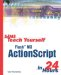# Workshop

 I l @ ve RuBoard

The quiz questions are designed to test your knowledge of the material covered in this hour . The answers to the questions follow.

#### Quiz

 1: What is the result of Math.min(10,Math.max(5,a)) when a is equal to 12? What is the purpose of such a statement? A1: The answer is 10. Math.max(5,a) resolves to 12; then Math.min(10,12) resolves to 10. The purpose of code like this is to make sure that the variable stays between two numbers . In this case, the result will never be less than 5 or greater than 10, no matter what "a" is. 2: If a movie clip is halfway around a circle, how do you get its horizontal position on the screen? A2: Use Math.cos with a value of 3.14, or Math.PI . Math.cos returns the horizontal position of a point on a circle. A complete circle is 6.28, or two times pi, so halfway would be 3.14 or pi. You also need to multiply the result by the radius of the circle. 3: What is the largest possible value returned by Math.random()*7 ? A3: The largest value of Math.random is slightly less than 1.0. So the largest possible value of Math.random()*7 is slightly less than 7.0. 4: If you use duplicateMovieClip to create a copy of a movie clip instance, what happens to the original instance? A4: Nothing. It is still there and any script assigned to it is still active.
 I l @ ve RuBoardSams Teach Yourself Flash MX ActionScript in 24 Hours
ISBN: 0672323850
EAN: 2147483647
Year: 2002
Pages: 272

flylib.com © 2008-2017.
If you may any questions please contact us: flylib@qtcs.net